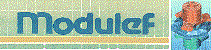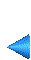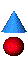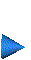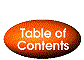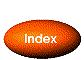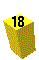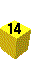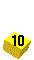## 1.5.8 Internal data structure

We use a system of lists with two elements, which we call CAR and CDR. The empty address is NIL=0 (parameter). In the following pictures, CAR will always be on the left and CDR on the right.

 CAR CDR

The free atoms are in a list of free atoms. The beginning of this list is FREE. Free atoms are linked through their CDR.

### Description of the DB for the CONSTRUCTION application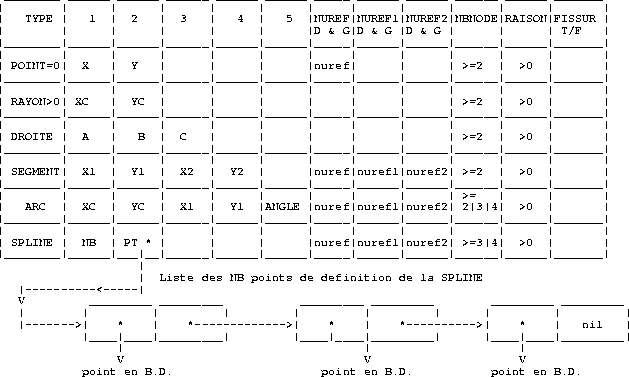Figure 1.44: Description of the DB for the CONSTRUCTION application

Figure 1.44 describes the DB for the CONSTRUCTION application with,

TYPE
the type of DB element
• POINT = 0. X,Y POINT.
• RADIUS > 0. CIRCLE of center XC,YC and radius RADIUS.
• LINE = -1. STRAIGHT LINE of equation A*x + B*y + C =0
with A*A + B*B = 1
• SEGMENT = -3. SEGMENT going from point X1,Y1 to point X2,Y2.
• ARC = -2. ARC of center XC,YC passing through point X1,Y1
and with an angle ANGLE from this point.
• SPLINE = -4. SPLINE.
• EMPTY = -1000. empty element.
for SPLINE,
• NB is the number of definition points.
• PT is the pointer on the list of definition POINTS of the SPLINE.
• NUREF is the reference number of the element (ARC or SEGMENT). Default: NUREF=0.
• NUREF1 is the reference number of extremity 1 of the element (ARC or SEGMENT). Default: NUREF1=0.
• NUREF2 is the reference number of extremity 2 of the element (ARC or SEGMENT). Default: NUREF2=0.
• NBNODE is the number of intermediate points in the element (extremities are included). Default: NBNODE=2.
• RATIO is the ratio of the geometric progression used to distribute the NBNODE-2 points from extremity 1 to extremity 2. Default: RATIO=1.

### Description of the DB for the PREP MESH application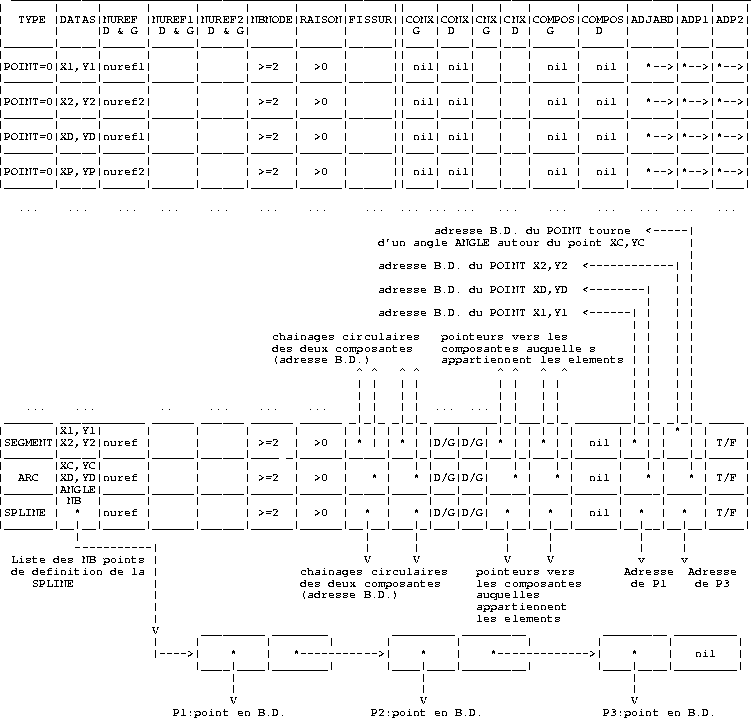Figure 1.45: Description of the DB for the PREP MESH application

Figure 1.45 describes the DB for the PREP MESH application, where

TYPE
is the type of DB element
• POINT = 0. X,Y POINT.
• SEGMENT = -3. SEGMENT going from point X1,Y1 to point X2,Y2.
• ARC = -2. ARC of center XC,YC passing through point X1,Y1 and with an angle ANGLE from this point.
• SPLINE = -4. SPLINE.
• EMPTY = -1000. empty element.

NUREF
is the reference number of the element (ARC or SEGMENT).
DB pointer on the POINT extremity 1 of the element (If the element is not cracked, NUREF of this POINT contains the reference number of extremity 1 of the element).
DB pointer on the POINT extremity 2 of the element or on the last POINT of the SPLINE. (If the element is not cracked, NUREF of this POINT contains the reference number of extremity 2 of the element).
NBNODE
is the number of intermediate points in the element (extremities are included).
RATIO
is the ratio of the geometric progression used to distribute the NBNODE-2 points from extremity 1 to extremity 2.
CONX(2)
DB pointers, used for the circular chaining of the elements of a connex component. Given one element, there are two and only two connex component that contain this element: the left component and the right component (left and right are relative to the orientation of the element). There are thus two chainings denoted by CONXG and CONXD.
CNX(2)
indicates which CONX is to be used for the next element (CONXG or CONXD) depending on whether CNX equals LEFT or RIGHT (LEFT=1, RIGHT=2)(parameter).
COMPOS(2)
inverse pointers to the two components to which the element belongs. CNXG is associated with CONXG and COMPOSG (exist only for ARCS and SEGMENTS). CNXD is associated with CONXD and COMPOSD (exist only for ARCS and SEGMENTS).
Beginning of the list of elements passing through the point (exists only for POINTS). (See the description of this list below).

#### Description of the list of elements passing through point i

The beginning of the list of elements passing through a point is ADJABD.
Figure 1.46 describes the list of elements passing through point i.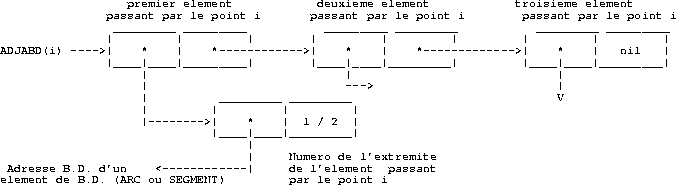Figure 1.46: Description of the list of elements passing through point i

#### Description of the list of components

The beginning of the list of components is COMP.
Figure 1.47 describes the list of components.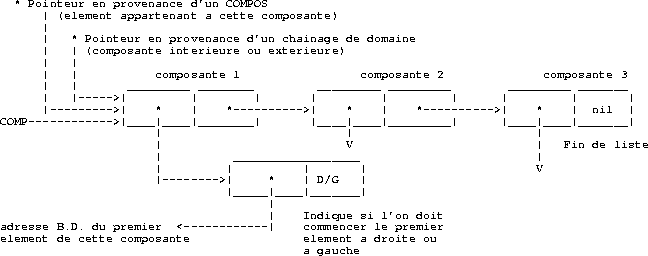Figure 1.47: Description of the list of components

#### Description of the list of domains

The beginning of the list of domains is SDOMN.
Figure 1.48 describes the list of domains.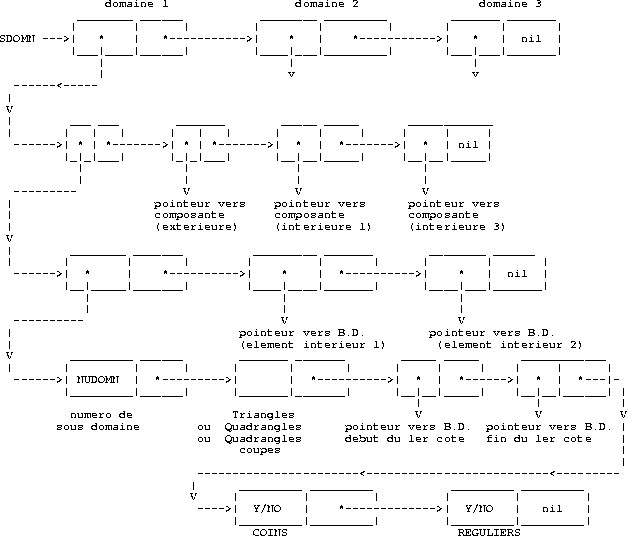Figure 1.48: Description of the list of domains

### Description of the DB for the EDIT_MESH application

The DB is the same as in the PREP_MESH application and the data structure DS_MESH  is as follows:

nbs
is the number of the last vertex
nbt
is the number of the last triangle
nba
is the number of edges that lie on curves (segment, arc, spline) that we will call boundary edges
nbsd
is the number of subdomains
nbsrft
is the number of vertices that are referenced by the elements
nbtria
is the number of triangles
is the number of quadrangles
nbtrou
is the number of holes in the mesh
freetr
is the beginning of the list of detroyed triangles
finbd3
is the number of the last DB element of PREP_MESH
The vertices
are defined in the following four parallel arrays:
cr(1:2,nbs)
is the array of vertex coordinates.
nsorig(1:nbs)
is the array giving the original vertex number of vertices that lie on a crack. The actual coordinates of vertex i are cr(1:2,nsorig(i)), while coordinates cr(1:2,i) are only used to visualize the crack.

Note: if vertex i is not on a crack, then nsorig(i) = i.

abcurv(1:nbs)
is:
• 0 if the vertex is not on a curve (segment, arc, spline), i.e. is an internal vertex of a subdomain,
• the abscissa of the point on a curve if this vertex is not an extremity of the curve, 0 if this vertex is the extremity of a curve. Note: curves are always parametrized between 0 and 1.
refs(1:nbs)
is the array giving the DB number of the element (point, segment, arc, spline) that supports the vertex, if it exists, or 0 otherwise.

Note: if the vertex is the extremity of a curve then the element in question will be the point extremity of the curve (cf. ADP1 and ADP2 (Appendix 1.5.8))

The finite elements
are triangles or quadrangles, which are internally represented with triangles whose edges are visible or not. This is used to draw the quadrangles. The arrays that define the triangles are:
nsea(1:6,nbt)
gives for each triangle the vertices and the adjacent triangles or boundaries edges. If nsea(1,ie)< 1 then triangle ie does not exist and reft(ie) gives the next destroyed triangle. Otherwise, triangle ie exists and
• nsea(1:3,ie) gives for each triangle the vertex numbers counterclocwise.
•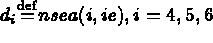, the 3 edges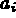of the triangle numbered from 4 to 6 have as vertices nsorig(nsea(i-3,ie)) and (nsorig(nsea(mod(i,3)+1,ie)).
• if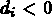the edge is a boundary edge. It is defined in aretdb with number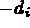,
• otherwise the edge is internal and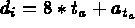where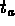is the number of the triangle adjacent to the edge and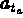is the number of the edge in.
anovue(1:nbt)
gives the visibility of the 3 edges for each triangle. If anovue(i) equals
0
all the edges of triangle i are visible,
1
only edge 4 of triangle i is not visible,
2
only edge 5 of triangle i is not visible,
3
only edge 6 of triangle i is not visible.

reft(1:nbt)
is:
• the array of the numbers of subdomain that contain the triangle,
• or the array of the chaining of triangles in a subdomain. reft(ie) gives the next triangle in the subdomain,

if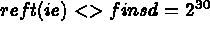, see also the tetdt array for the beginnings of lists of subdomains.

The boundary edges
of the mesh are defined as follows:
aretbd(1:2,nba)
gives for each edge its two vertex numbers,
: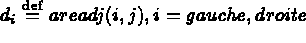same definition as nsea,
refa(nba)
gives the DB address of the support of the edge, which always exists by definition.

The subdomains
are defined in the following arrays:
refsd(nbsd)
gives the reference numbers of subdomains, if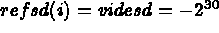then the subdomain does not exist.
trfsd(3,3,i)
gives the transformation applied to subdomain ptorsd(i) to obtain this subdomain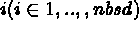.
strfsd(i)
gives the sign of the transformation (for example: -1 for a symmetry with respect to a straight line)
ptorsd(i)
gives the number of the original subdomain (if ptorsd(i)=i then subdomain i is not transformed (original subdomain))
tetsd(nbsd)
is an array that gives for each subdomain the first triangle of the list of triangles of this subdomain (cf. reft)

### Description of AM, AM_FMT, AMDBA meshes

The mesh is only composed of triangles and can be defined with the help of the following two integers and four arrays:

nbt
is the number of triangles
nbs
is the number of vertices
nu(1:3,1:nbt)
is an integer array giving the three vertex numbers counterclockwise for each triangle.

c(1:2,nbs)
is a real array giving the 2 coordinates of each vertex.
refs(nbs)
is an integer array giving the reference numbers of the vertices.
reft(nbs)
is an integer array giving the reference numbers of the triangles.

Note: the vertices that are not referenced by a triangle will be eliminated. In this case the vertex numbering will be compressed accordingly.

#### AM files

xxx.am files are read as follows:

```     open(1,file='xxx.am',form='unformatted',status='old')
+                 ((nu(i,j),i=1,3),j=1,nbt)
+                ,((c(i,j),i=1,2),j=1,nbs)
+                ,( reft(i),i=1,nbt)
+                ,( refs(i),i=1,nbs)

close(1)
```

#### AM_FMT files

xxx.am_fmt files are read as follows:

```     open(1,file='xxx.am_fmt',form='formatted',status='old')
read (1,*)  ( reft(i),i=1,nbt)
read (1,*)  ( refs(i),i=1,nbs)
close(1)
```

#### AMDBA files

xxx.amdba files are read as follows:

```     open(1,file='xxx.am_fmt',form='formatted',status='old')